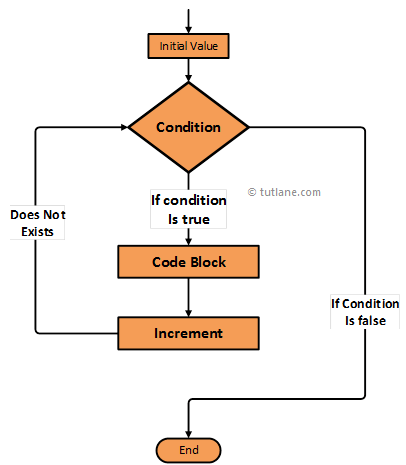# Swift For Loop

In swift, for loop is used to iterate through the sequence of items in an array or list or collection to execute specified statements repeatedly based on the defined conditions.

If we get requirements like need to execute a set of statements repeatedly based on the specified number of times then by using for loop we can easily achieve this functionality in a swift programming language.

Now we will see the functionality of for loop in a swift programming language using an algorithm diagram.

## Swift For Loop Flow Diagram

Following is the swift for loop statement flow diagram to represent how the functionality of for loop will work in a swift programming language.If you observe above swift for loop flow diagram first we need to declare and initial the value based on that we will start looping repeatedly till that condition satisfies.

In case if the defined condition is true then it will execute the statements inside of that condition and it will increase the value of a variable which we declared initially and continue checking the condition to execute the statements till it fails.

## Syntax of Swift For Loop

Following is the syntax of defining for loop in swift programming language.

for <INITIAL VALUE >; <LOOP CONDITION>; <INCREMENT>

{

}

If you observe above for loop syntax we defined multiple values those are

Initial Value – We need to declare and define initial value based on that value we will start checking the condition.

Loop Condition – We need to define the condition and that needs to be satisified to execute the statements inside of that condition.

Increment – After completetion of executing the for loop body statements it will jump back to Increment statement to increase the variable value which we defined initially and continue evaluating the conditions till the condition fails.

Now we will see how to use for loop in a swift programming language with examples.

## Swift For Loop Example

Following is the simple example using for loop in swift to loop through the list of items.

let counter = 3

var listarr:[Int] = [1, 2, 3]

var sum1 = 0

for var i = 1; i <= counter; i += 1 {

print(listarr[i])

}

If you observe above for loop example we declared and defined a variable “i” and checking the condition whether defined variable value less that equal or not based on that we are printing the list of items repeatedly till the condition fails.

When we execute above program in swift playground we will get a result like as shown below.

1

2

3

This is how we can use for loop in a swift programming language to execute defined statements continuously based on our requirements.